# Pixel Recursive Super Resolution

Google released a method for recovering pictures from low resolution to high resolution using pixel recursive super resolution model.

Paper source: https://arxiv.org/pdf/1702.00783.pdf
Related reports: https://news.ycombinator.com/item?id=13583476

At each level of the pyramid, a separate generative convnet model is trained using the Generative Adversarial Nets (GAN) approach (Goodfellow et al.). Samples drawn from our model are of significantly higher quality than alternate approaches.

Recently, Google released a method for recovering pictures from low resolution to high resolution. Compared with state-of-art approaches, this paper proposed an End-to-End framework to accomplish the super resolution task as a whole. It is comprised of two convolutional neural networks, one being a prior network that describe the skeleton of low resolution images, the second being a conditioning network to optimize detailed features. This specific method highlights the improvement on detailed features recovery for high resolution images and promote the theory in a probabilistic paradigm. Specific to the core algorithm, I will provide a review on the sampling strategy, model architecture, and approach analysis pieces.## Introduction & Related work

The implication of the Super Resolution Problem lies in recovering the image’s resolution. Heuristic solution manages to repair and complement detail patches to reconstruct high-resolution picture. However, for missing features that do not exist in the original images, the recovery model is required to generate them naturally. Hence optimal super resolution restore model must account for the complex variations of objects, viewpoints, illumination and occlusions. It should also be capable of drawing sharp edges and making hard decisions about the type of textures, shapes, and patterns presentation in different parts of images. According to the stated requirement above, there is significant difficulty in generating visually vivid high resolution images.

Since the super resolution problem has a long history in computer vision research. There are diverse feasible methods to recover high resolution images. An interpolation heuristic approach is easy to implement and widely used, basically it takes the strategy that takes the average among all plausible detailed feature values. However, the drawbacks of these similar methods are also obvious, because the linear models cannot properly express complicated dependencies between the input information and output results, thus the resulting images tends to be blurry.

In order to obtain plausible vivid image details, detailed de-blurr approach has been proposed. The approach is similar to dictionary construction(a rather primary and simple approach), further study is learning layers of filter kernels to extract multiple abstractions (features existed in CNN layers) in CNN, then to adjust network weights by measuring pixel-wise loss between interpolated low-resolution images and high resolution images. Basically, this multi-layer filter based approach performs better with more feature layers. Thus, the SRResNet achieves the expected performance by learning from many ResNet blocks. This paper applies a similar conditional network design for better processing high-frequency features.

Beside the design of high-frequency features simulation, the following proposed super resolution network model is named PixelCNN. PixelCNN aims to generate prior knowledge between low resolution and high resolution images. Comparing with another generative model – GAN, both of them will suffer when training samples lack diversity in distribution. PixelCNN is more robust to hyper-parameter changes. But GAN is rather fragile, only repeat sufficient learning epochs to fool a non-stationary discriminator, such strategies are rather tricky, thus we have the embryo of Google super resolution network. Referred to in the following figure: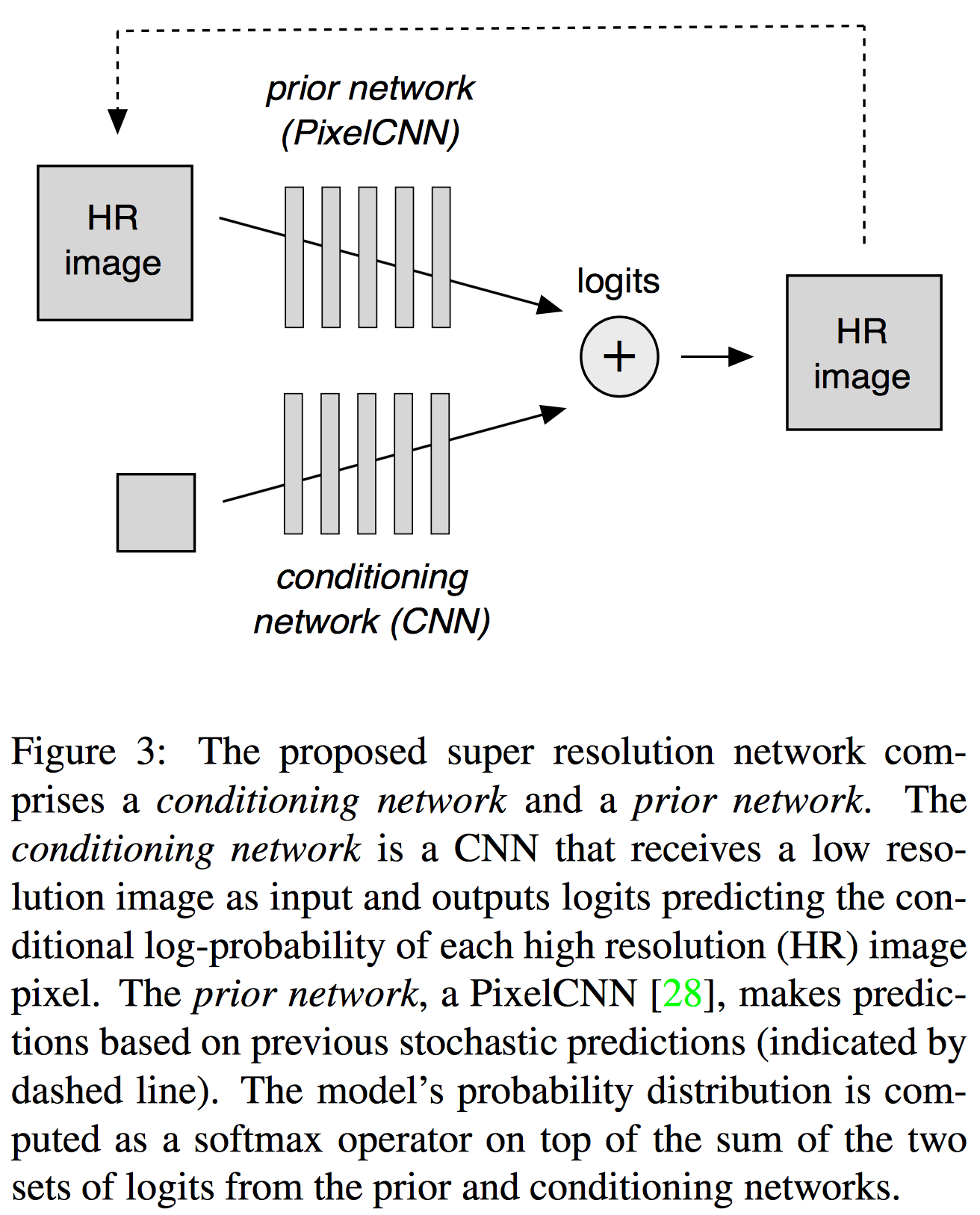The end-to-end model is established by two network component:

1. Prior network using pixelCNN

2. Conditioning network using Res blocks from ResNet.

## Proposed Approach and Experiments

In the following section, this paper illustrates the superiority of pixel recursive super resolution framework and the potential issues with pixel independent super resolution, then validate the theories on corresponding dataset, comparing self-defined metrics.

To state the necessity for deploying recursive super resolution method, this paper first illustrated the theory of pixel independent super resolution method> The next section demonstrates such theory will fail in conditional image modelling. The unsatisfactory experimental results led to Google’s recursive model.

The basic workflow is shown as:In order to model super resolution problem, the probabilistic goal is to learn a parametric model of: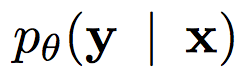where x and y denote the low resolution and high resolution images respectively. Once we obtain the conditioning probabilities of pixel values, it’s possible to reconstruct whole picture with high resolution. We can apply the above setting in a dataset, where y* denotes the ground-truth high resolution image, thus formulating the object function mathematically. The optimization goal is to maximize the conditional log-likelihood objective as follows :The key factor is to construct the most suitable distribution of output pixel values. Hence we are able to recover most vivid high resolution images with sharp details.

●  Pixel independent super resolution

The naïve way is assuming every prediction pixel value y is conditionally independent from others, therefore overall probabilities p(y|x) is product by multiplying each individual predictions.

Suppose one given RGB image has three color channels, and each channel owns M pixels. Applying log function on both side will yield: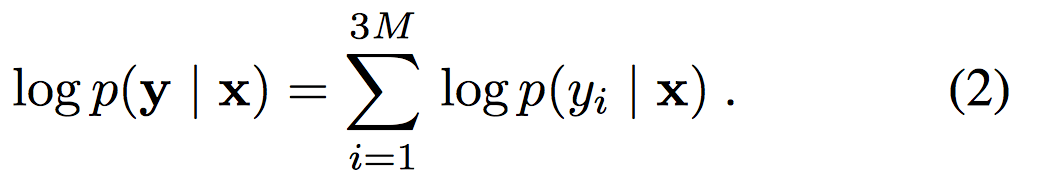If we assume the predicted output value y as a continuous value, then formula (2) could be reconstructed under Gaussian distribution model as :Where y_i denotes the non-linear mapping output via Convolutional Neural Network model,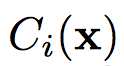denotes the estimated mean for the ith output pixel,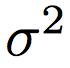denotes the variance, generally variance is known before instead of learning obtained, thus the only learned term is the L2 norm between mean value and individual prediction. Then maximization of log-likelihood (expressed in (1) ) could be transferred as minimization procedure of MSE(Mean Square Error) between y and C. In the end, the CNN is able to learn a set of Gaussian parameters to obtain optimal mean value C.

For continuous values, we apply Gaussian model, for discrete values, we use multinomial distribution to simulate the distribution( dataset noted as D ), then the prediction probability could be described as: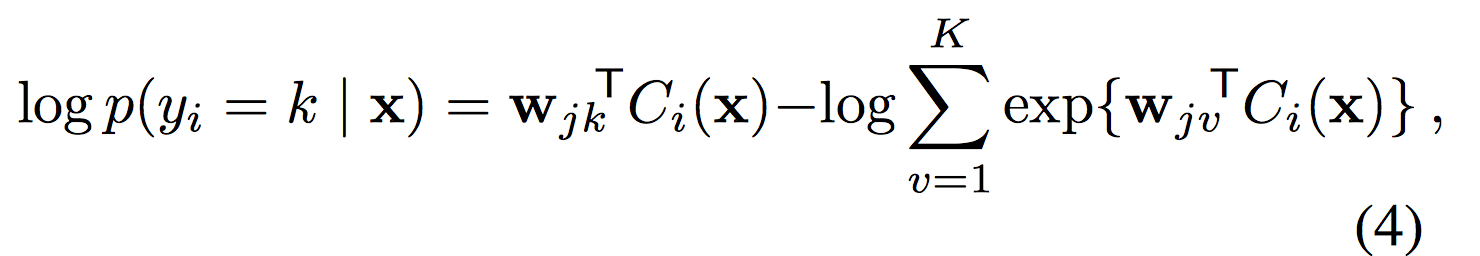Then our objective is learning to obtain optimal softmax weights values from predictive models, whereare softmax weights of K possible discrete pixel values under three channels.

However, the paper claims the independent models illustrated above is incapable of dealing with multi-modality occasion, hence its performance is inferior to the multi-modal capable method in some specific tasks, eg. colorization, super resolution. Then it follows an experimental demonstration on MNIST corner dataset (the digits figure are identical only for locating objects either in top-left corner or bottom-right corner),Refer to MINIST experiment figure, the digit generation results under different methods shows the difference. Pixel-wise cross-entropy method can capture crispy images, but fail to capture stochastic bimodality, thus the digits object appeared at both corner. A similar situation happens on L2 Regression method. The final results give two blurred digits in one output high resolution image. Only pixelCNN can both capture the location information and crisp image information, hence it further illustrate the superiority of using pixelCNN in the proposed model.
●   Pixel recursive super resolution

After the paper demonstrate that the pixel independent super resolution method has limitations, it gradually leads to interpretation of its proposed approach – pixel recursive super resolution. The novel approach theory still aims to maximize the log-likelihood of given samples x. Recursive model part assumes conditional dependency exsisted between output pixels . In the end, in order to approximate this joint distribution, the recursive approach factorize the conditional distribution using chain rule asWhere each generated output is conditionally depended on the input and previous output pixels, forThen the proposed pixel recursive super resolution framework (refer to previous architecture ) embedded CNN can be illustrated as:With an input x, let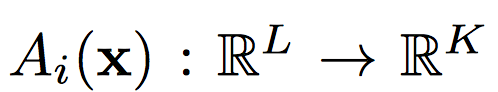Denote a conditioning network predicting a vector of logit values corresponding to the K possible values that the i th output pixel can take.

Similarly, let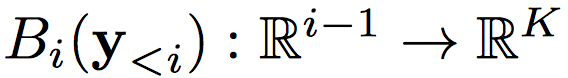Denote a prior network predicting a vector of logit values for the i th output pixel.

According to the paper, it optimizes both two networks jointly, updating and obtain the maximal log-likelihood via stochastic gradient ascend method. That is, optimize a cross-entropy loss between the model’s predictions in (6) and discrete ground truth labelsThen the cost function is:Where lse(·) is the log-sum-exp operator corresponding to the log of the denominator of a softmax, and 1[k] denotes a K-dimensional one-hot indicator vector with its k th dimension set to 1.

However, when deploying the cost function in experiments, the model trained tend to ignore conditioning network. Thus the paradigm includes a new loss term to measure the cross-entropy between the prediction and ground truth labels in conditioning network, noted by: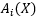We obtain the new formula as :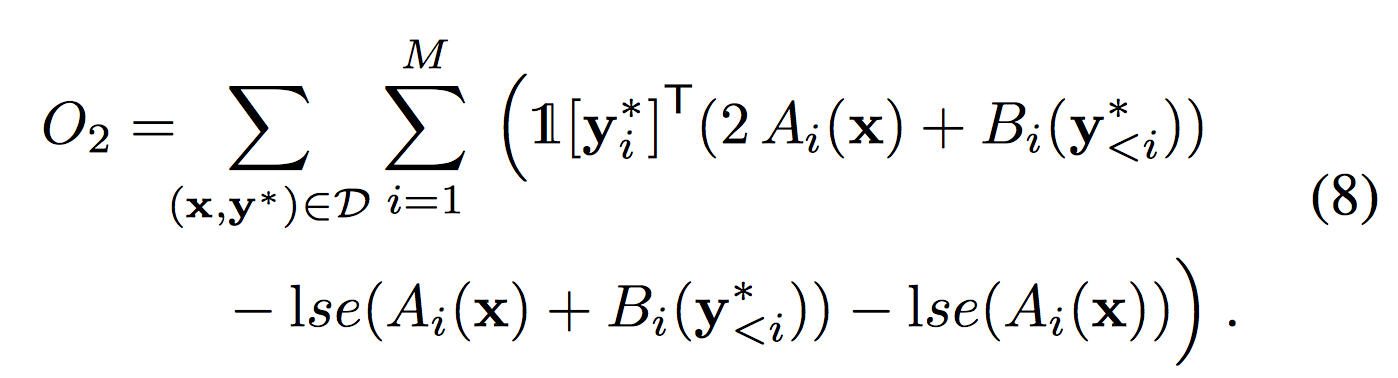Besides the training paradigm definition, in order to control the concentration of sampling distribution, additional temperature parameter is added, which infers to greedy decoding mechanism: always choose the pixel value with the largest probability and sampling from a tempered softmax. Thus the distribution p is adjusted as :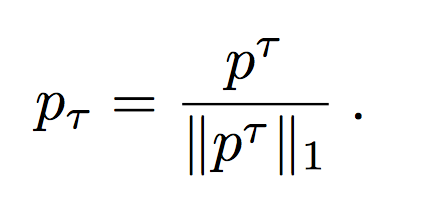With all the theoretical preparations down, the paper goes onto the implementation phase. The experiments are accomplished under TensorFlow framework, utilizing 8 GPUs along with SGD updates. For the conditioning network, a feed-forward convolutional neural network is built. It takes an 8×8 RGB image through a series of ResNet blocks and transpose convolution layers, while maintaining 32 channels throughout. The last layer uses a 1×1 convolution to increase the channels to 256×3, and uses the resulting activation to predict a multinomial distribution over 256 possible sub-pixel values via a Softmax operator.

As for prior network, typically pixelCNN, the experiments used 20 gated PixelCNN layers with 32 channels at each layer .

●  Results and evaluation

To validate the performance of the proposed framework, the paper chose to recover two types of objects: human faces and bedroom scene. All training images are from CelebA dataset and LSUN Bedrooms dataset. To satisfy the requirements of network input, the authors also applied a necessary pre-processing stage to each dataset: cropped celebrity faces from CelebA dataset and cropped central images for LSUN Bedrooms dataset. In both datasets, they also resized the images to 32×32 with bicubic interpolation, and again to 8×8, constituting the output and input pairs for training and evaluation phase.

After training, the recursive model is compared with two baseline network: a pixel independent L2 regression (“Regression”) and a nearest neighbors search (“NN“) . The visual results are given by following figure:According to visually judging the results between different methods, NN method can give crisp images but with rather large gap to ground truth, the regression model gives a close rough sketch, and the pixel recursive super resolution approach lies seemingly in the middle between the two baseline methods. It captured some detailed information, but not significantly better performing than other methods.

Additionally, given the different temperature parameter, there are slight differences shown in the following figure:For measuring performance, it quantifies the prediction accuracy of trained model compared to ground truth using pSNR and MS-SSIM (Table 1), besides the traditional measurement, the result also involved a human recognition study.According to the result table, the recursive model didn’t perform well on the pSNR and SSIM measurement. Although the paper also cited the other two measurements to state validation. However, it currently seems to lack strong convince when adding synthesized information, or may need further work to test the measurement validation. For human fooled percentage, the model performs superior than other methods, hence one could say the generated images are more photo realistic.

In summary, the pixel recursive super resolution method proposed an innovative framework to balance the trade-off between rough skeleton and details capture. However, personally I think the outcome performance is not very convincing to state the model is really productive in recovering low resolution images to high resolution. Although the different resolution setting made it difficult to compare, it did achieve good performance for human judgement. Thus, it can potentially have good industrial application in the future.

Analyst: Angulia Yang | Editor: Hao Wang| Localized by Synced Global Team : Xiang Chen